## //----------------------------// ArtMatic 4 in 2 out components //----------------------------//

Introduction
Complex multiply Z*C
Complex divide Z/C
Complex power Z^n + C
Complex Z^2 + C
Complex Z^3 + C
Complex C(Z+ 1/Z)
Complex C exp(Z)
Complex C sin Z
ComplexMap 4D
(xziy) City Light & Ref #
2D Vector Maths #
Interpolate
Distance divide
Exp field
Escher disks
Mondrian displace
Facet Mix
Moebius(Z) + C
3D Motion Path render
Logic tools #
Min <-> Max
Blended Max
Abs & minimum
Y compare
Split space
Distance compare
Split Disk
Alternate Split
Split 4
x from Z, y from C
42 Compiled tree

## Introduction

These components serve a broad number of uses and are often 2D vector functions that operates on two 2D input vectors. Many of these components are mathematical primitives for performing complex number arithmetic. The complex number inputs are called Z and C; Z refers to the two leftmost inputs, x and y, where x is the real part and y is the imaginary part of Z=x+iy. Likewise, C is made up of the two rightmost inputs C=z+ iw.
An essential in-depth discussion of ArtMatic structures Trees and components is found in ArtMatic Designer References and in Building trees.

##42 Complex add Z+C

parameters :
A : Scale (0.50 : 2.)

discussion :
Returns the Complex addition of Z(x+iy) and C(z+iw) scaled by 'Scale' if not 1.

##42 Complex add N*Z+C

parameters :
A : N real part (-4. : 4.)
B : N imaginary part (-4. : 4.)

discussion :
Parameters A (Real Part) and B (Imaginary Party) define the real and imaginary parts of complex number N that multiplies incoming Z (x+iy). C (z+iw) is added to the result. The result is the complex (x*A) - (y*B) +z, (x*B) + (y*A)+w which is simply the 2D coordinates in the plane (x,y)

##42 Complex multiply Z*C

parameters :
No parameters.

discussion :
This returns the complex multiplication of Z and C. The real part of the product is sent out the left outlet and the imaginary part is sent out the right. The result is the complex (x*z - y*w , x*w + y*z)

parameters :
No parameters.

##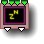42 Complex power Z^n + C

parameters :
A : n power exponent (-8. : 8.)

discussion :
Raises the complex number Z (x + iy) to the power of n and adds C (z + iw) and n is the value of parameter 'power exponent'. Like the other complex number primitives, this component is handy for creating a new space with aspects of the spaces that feed it. It returns a single transform and has no recursion parameter. Advanced users will find this primitive useful for implementing their own formulas. Note that with n=2 we have the same function as the Mandelbrot set below.

##42 Complex Z^2 + C

parameters :
A : Recursions # (0 : 128)
B : Outer Slope (-2. : 2.)

discussion :
The Mandelbrot/Julia Set equation implemented as a space mixing/distortion component. This component will usually be used near the top of the tree and takes two spaces as input, treating them as complex numbers Z and C where the first input of each pair is the real part of the number and the second input of each part the imaginary part.

For Julia Sets connect the C (z+iw) inputs to a
12 constant tile to get fixed parameter C values.

For Mandelbrot set connect the Z to a constant (0,0) and C to xy space as the Mandelbrot set is a Map of a variable parameter C effect on escaping orbits. Interestingly the nicest Julia sets are found when the parameter C lies at the boundaries of the Mandelbrot set.

The output of the component is a transformed complex. When the recursion escape to infinity at the exterior of the sets the coordinates are transformed to be still usable in further shading. The "Outer Slope" parameters affects the curvature and scale of the "outer" regions of the sets.

See also the distance estimation rendering of Mandelbrot set in the 41 tile DEM Mandelbrot(U*U+V)

##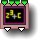42 Complex Z^3 + C

parameters :
A : Recursions # (0 : 128)
B : Outer Slope (-2. : 2.)
C : Outer offset (0. : 1.)

discussion :
This is the cubed version of the Mandelbrot equation : Z^3 + C

##42 Complex C(Z+ 1/Z)

parameters :
A : Recursions # (0 : 128)

discussion :
This equation involves both Z and its inverse. The complex inversion maps 0 to infinity and infinity to zero. When recursed with adequate C constants it will generate fascinating space filling fractals. This can also be used as a building block in compiled trees for creating new fractals.

Example: Complex C(Z+ 1/Z) using (-0.06, 0.92) for C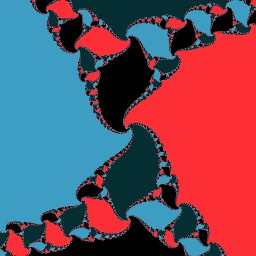##42 Complex C exp(Z)

parameters :
A : Recursions # (0 : 64)

discussion :
Returns C * exp(Z) in the complex plane . The complex exponential function is given exp(Z) =e^x cos(y)+ i e^x + i sin(y) with Z=x+iy. This component, CPU-intensive, will create amazing fractals when recursed. In general you will pass a non zero constant vector in C(z,w).

Example: C exp(Z ) using (0.4901, 0.1982) for C with recursion at 36. White zone are escaping to infinity very fast.##42 Complex C sin Z

parameters :
A : Recursions # (0 : 128)
B : Outer Slope (-2. : 2.)

discussion :

discussion :
Returns C * sin(Z) in the complex plane.The complex implementation of sin(Z) uses hyperbolic sines namely sin(Z) = sin(x)*cosh(y)+ i cos(x)*sinh(y) with Z=x+iy. The recursion parameter allows to loop the transform to create a 2D fractals sin . In general you will pass a constant non zero vector in C.

Example: C sin Z using (0.4277, 0.0945) for C##42 ComplexMap 4D

parameters :
A : Real (-2. : 2.)
B : Imaginary (-2. : 2.)

discussion :
Like the 2D Complex map, this component is a complex number polynomial. This version is recursive and is sensitive to the preference for Max iterations for fractals. This component creates particularly rich output.

##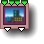42 (xziy) City Light & Ref #

parameters :
Algorithm slider : (0 : 7)
B : Amount (0. : 2.)
C : Frequency (0. : 8.)

discussion :
Like the related 32 component, this component provides lighting and reflection information for terrain-based xziy cities buildings' windows.
44 xziy City Textures is the companion component that provides the color texture for the buildings (usually provided by 24 City Maps ). The pattern (map) name should be the same for both the City Textures and 32 City Light & Reflection #, and the frequencies should be the same as well.

Inputs. When discussing the inputs to this component, the inputs are referred to as (from left to right): x, z, i, and y. The left two inputs (x and z) are the ground co-ordinates (latitude & longitude), 'i' is the texture index which is normally received from 24 City Maps, and y is elevation.

Texture index. Texture index is usually provided by 24 City Maps but you can pass in a constant to select a particular building texture or manipulate the index to select different textures for the same buildings.

Outputs. The left and right output values (also called x and y) are the illumination level and reflection level. Normally, these will be mapped to Ambient Light and Reflection in ArtMatic Voyager (using Voyager's extra output mapping options).

Parameter A selects the city/building map to use. The names correspond to the maps created by the 44 City Textures component with which this component is meant to be used. For perfect sync, use same frequency and texture index (i) that is passed to 44 City Textures. The maps are: City Texture A, City Texture B, City Texture B, City Texture C, City Texture C, City Classic

algorithms :
• City Texture A # :
• City Texture B # :
• City Texture C # :
• City Classic # :

##42 Add normalized

parameters :
A : Scale (-2. : 2.)
B : Normalize (0. : 1.)

discussion :
Another useful mathematical primitive that is often used for implementing systems based on differential equations. The component's formula is Z (xy) + (C(zw)/N) where N is the magnitude or length of C (square root of x^2 + y^2).

##42 2D Vector Maths #

parameters :
A : Amplitude (-2. : 2.)

discussion :
This component provides several vector math primitives. The inputs are treated as two sets of vectors: U (x,y)( inputs 1 2) and V (z,w) ( inputs 3 4) to which the selected operations are applied. This component can create interesting composite spaces.

The output algorithm is indicated by the name.

algorithms :
• vec Subtract :
• vec Multiply :
• vec Divide :
• line Normale :
"Line Normale" returns in x y the normalized line equation coefficient from input points.

##42 Interpolate

parameters :
A : interpolate (0. : 1.)

##42 Radial Interpolate

parameters :
A : Amplitude (0.12 : 4.)

discussion :
This component interpolates from space A(xy) to space B(zw) according to distance from the origin in space A.

##42 Distance divide

parameters :
A : Amount (-1. : 1.)
B : Phase (-8. : 8.)

discussion :
This component divides the first space by the distance of the second pair of inputs from the origin. It can have perspective-like effects. Because division is used, the results change if the order of the inputs changes. Phase adds an offset to the distance value. Amount is an interpolation between the distance and 1. When the amount is zero, the distance is ignored.

Distance divide can create interesting enclosed spaces from infinite spaces. When two infinite space transforms are passed in, the result tends to be a complex warped space enclosed in a disk or figure eight-like shape. The results can be quite complex from even the simplest trees. When using this component, be sure to carefully explore the results when the Amount parameter is close to zero. When enclosed spaces are passed in, the result is sometimes an infinite unfolding of the enclosed space. The order of the space transforms matters (i.e. A/B is not the same as B/A). It can be interesting to explore what happens when the same 2-output tile feeds both the left two and right two inputs. The examples below use Interlaced Grid after Distance Divide, as it helps visualize the space transform. You may want to use similar trees to become familiar with this transform. (If reading this manual on a computer screen, be sure to zoom in to see details that might be missed onscreen).

##42 Exp field

parameters :
A : warp (0.05 : 0.95)
B : Amplitude (1. : 4.)

discussion :
This component creates a composite space that has aspects of both input spaces. A field equation is applied to both pairs of inputs and the results are added together. The field equation is similar to a gaussian curve and hence the maximum output is found where the input value is 0 and approaches zero as the input reaches infinity.

##42 Escher disks

parameters :
A : Offset x (0. : 1.)
B : Offset y (0. : 1.)
C : Radius (0.50 : 2.)

discussion :
A complex space distortion function which tiles space with disks using the Mandelbrot set equation. The C input (the second input pair) act as the C constant in the Mandelbrot equation.

##42 Mondrian displace

parameters :
A : Displacement (0. : 8.)
B : Phase (-16. : 16.)
C : Frequency (0. : 4.)

discussion :
A displacement map for creating Mondrian-like effects. The displacement uses a random arrangement of discrete rectangular zones. The two rightmost inputs control the pattern's space that displaces the input (x,y) space.

##42 Facet Mix

parameters :
A : Amplitude (0.12 : 4.)
B : Phase (-8. : 8.)
C : Frequency (0.12 : 2.)

discussion :
This component generates a composite space by randomly choosing facets/bubbles from one space or the other so that the resulting space has a mix of bubbles from both spaces. In this way, chunks of space B become mixed with space A. Amplitude is a zoom factor applied to the inputs before feeding them into the bubble algorithm. Phase is an offset factor. Frequency determines the "bubble" size.

##42 Moebius(Z) + C

parameters :
A : Angle (-6.28 : 6.28)
B : Scale (-4. : 4.)
C : Imaginary (-6.28 : 6.28)

discussion :
This component applies a
Moebius complex transform to the space provided in the first two inputs and adds the space provided by the right inputs. This component can create very interesting warped spaces and fractal-like patterns from even simple inputs. See also the 22 Maths tools # that implements various flavors of the Moebius transform.

##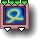42 3D Motion Path render

parameters :
A : Iterations (2 : 500)
B : step divide (1. : 256)
C : level/width (0. : 8)
D : Frequency (1/100. : 10)

discussion :
3D Motion Path render will render a large number of 3D points provided by a CT connected to input 4 like the scalar 41
3D Motion Path render version. Unlike the later it also outputs local space-time coordinates in first output, coordinates that can be used for shading and texturing and to further modulate the render.
Note that ArtMatic does automatically assume if main reference orientation is Y or Z depending on the extent of the set of points. The object orientation is modulated by the derivative so that the orientation follow the path naturally. Orientation is computed trying to keep "z" on the path direction, Y pointing up and X pointing sideways perpendiculary to the path.

inputs:
3D Motion Path render takes a 3D space in inputs (x,y,z) and the list of 3D points defining the path as a 3D or 4D packed vector in input 4 (The positions of 3D point has to be packed so they can be fed to the single input 4). The 3D points are provided by a 11 Compiled Tree connected to input 4. When the 4th input is a 4D vector the additional information can modulate the radius of each point. The CT that computes the points positions shall return the coordinates as a function of time only in the form of a parametric curve (X=F(t),Y=G(t),Z=H(t), and optionally Radius=I(t)).

outputs:
Additionally to the scalar version, this components outputs in the first outlet local coordinates for each points as a packed RGBA vector with the local time available in the alpha channel (xyzt). The DF field is returned in the second output.
The local coordinates will follow the path orientation and will typically be used for texturing, or as space coordinates to place another 3D DF objects. The Y axis is mapped to the path orientation. In the alpha of the packed xyzt will be a normalized parameter t that will go from 0 to 1 along the path. When 'Render as Spheres' is used t is the local time of each point divided by the point numbers and is discontinuous. Otherwise t is smoothed out to be as continuous as possible.
If you only need the scalar DF field use the 41 version at 3D Motion Path render

parameters:
The number of iteration will decide how many points are generated. When the CT is connected to time, points will move automatically. The 'step divide' controls the time resolution, hence the amount of time between two consecutive points. The lower the more points will be spaced. 'level/Width' parameter sets the size of the rendered discs or line. If the CT inputs is packed 4D the radius in the point data is added to the level. No global x or y inputs shall be used within the CT as the points are computed just once before each frame and x, y doesn't exist yet. Use 'Frequency' at 1 unless you want to enlarge or reduce the whole rendered shape.

The output is DF compatible when the input space (x,y) is unscaled, thus an immense variety of modeling possibilities opens with this new component for 3D rendering in ArtMatic Voyager.

The tile has several rendering algorithms that offers the following rendering options :

algorithms :
• Render as Spheres:
Each point is rendered as a DF sphere. Spheres are accumulated using the "union" logic. Packed output coordinates will hold the (xyzt) vector where t is the normalized time along the path, as in most modes exept "xyzw spheres".

•Spirale in xz with sine in y. 'level' set at 5

• Render as DF lines:
The list of point is rendered as DF lines.

•Spiraling line using 'DF lines' and 'level' set at 5

• as morphed spheres:
The list of point is rendered asDF spheres but they are accumulated using exponentials with the Morph algorithm explained in detail in the 11 Memory Maths "Morph" section. This mode will blend each sphere naturally and is an ideal choice for modeling clouds, smoke, fluid or organic shapes.Spirale-Snake using 'morphed spheres'. The texture is using the alpha of the packed xyza that hold a normalized (0-1) time value 't'

• as upward spheres:
Each point is rendered as a DF sphere like the first option but coordinates keeps the Y axis pointing upward. This mode is suited when instanciating trees or buildings to make sure Y stays vertical.

•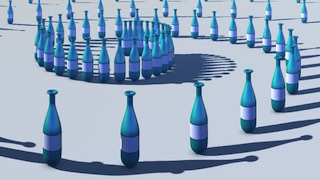Spiraling cluster bottles using 'upward spheres'.

• as xyzw spheres:
-This mode, only available when the CT outputs 4D vectors, is equivalent Render as Spheres but it outputs the full incoming xyzw data instead of time in 4th position of packed output 1. The w is recomputed to be continuous and can be used to scale textures or growth the DF field. In this mode the growth is not applied inside the integration to minimize problems with DF field coordinates.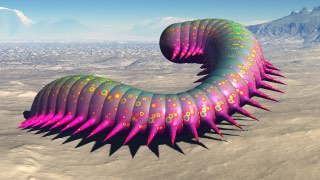Alien Worm using 'xyzw spheres'. The change of worm thickness is added aftwerwards from the w value.
Note the "legs" spreadig on the X axis in red.

(Voyager Examples/Components/DF Motion path/MPC3D AlienWorm.vy)

Note : Whatever the render mode is, the coordinates sent in out1 can be used to render another DF primitive. Here a XYZ Shapes # Roman arch is placed at each point of the path and added to the 3D line rendering.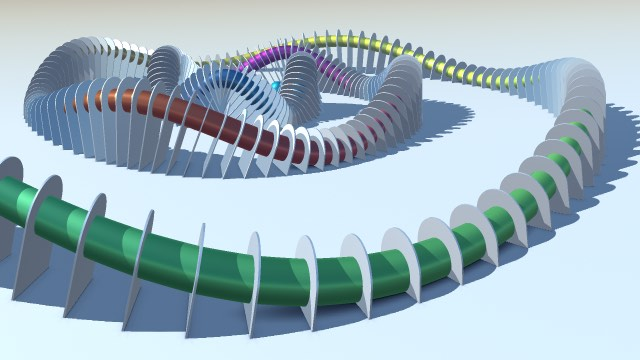Note :You may also use this component to instantiate efficiently a large number of objects like with of Motion Cluster or other Jittering tiles. In that case you will use the coordinates to place each object instance and combine the DF field of the Motion Path with the object own for an optimal convergence. The advantage is the full control of the placement of all instances given by the equation you enter in the CT.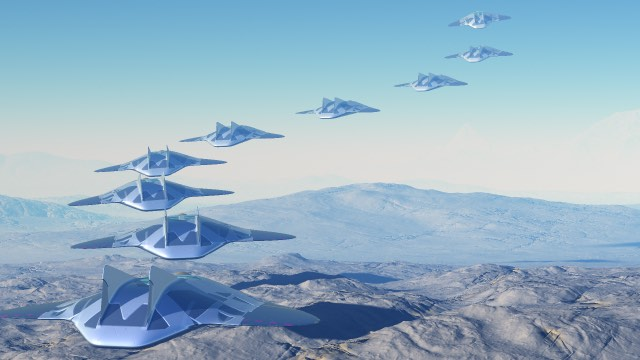Ships flying upward exponentially, example in Voyager Examples/MPC3D cluster X.vy

3D Examples : Voyager Examples/Components/DF Motion path/
2D Examples : Libraries/Components demo/Cosmic snake spirale

##42 Logic tools #

parameters :
B : Smoothness % (0. : 1.)

discussion :
With the introduction of dual DF components like DF Cities, DF Constructs & DF Polyhedron it became practical to have a logic operator that would handle dual streams of DF volumes. The exact nature of the double stream is unimportant but in general it would be edges or structural parts for architectural elements followed by another part that can be shaded differently like windows, reflective surfaces or a transparent volume.

42 Logic tools performs exactly that : perform logic operation between 2 dual DF object. Note the result is also a dual DF object meaning this component can be chained. It introduces also more subtle operator that treats the "structural part" and the "window part" slightly differently with the Struct series.

boolean operators.

Lets name the dual DF vector of Object A A(s,w) and Object B B(s,w) for the second. Then lets note union operator "U" (logical OR) and intersection operator "I" (logical AND) (as the inverted U symbol is not in ASCI). Algorithm Note: When structures are thin edges intersect or subtract will probably leave little left of them.

algorithms :
• Union:
(E U As U Bs , Aw U Bw) is the result.
• Intersect:
(E U (As I Bs), Aw I Bw) as the result.
• Subtract:
Performs the named boolean operation in parallel for the structure and window parts.
(E U (As - Bs) , Aw - Bw) is the result.
• Cubic smooth union:
• Cubic smooth intersect:
• Cubic smooth subtract:
• Edged union:Edged union: of a dodecahedron A and an hexagonal prism B. Notice the added edge at their intersect.

• Edged intersect:
• Edged subtract:
The Edge series will perform the edge calculations upon an intersection of A and B full volume (union of s and w). The "thickness" parameter sets the edge size. The rest follows the usual logic. E= Edge( As U Aw , Bs U Bw)Edged subtract: hexagonal prism was removed but the edge is still there

• Struct intersect :
Unlike the parallel straight Intersect, Struct intersects keeps the struct parts of A minus the Bw volume; The "window" parts behave like in normal intersect.
(As U (Bs -Bw) , Aw I Bw) is the result.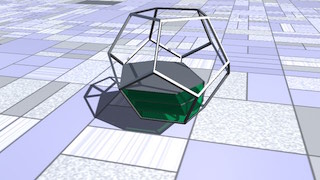Common w parts and A struct are preserved. B structs are preserved only when inside Aw volume.

• Struct subtract :
Unlike the parallel subtract, Struct subtract keeps A struct intact and B struct when inside A.
(As U (Bs I Aw) , Aw - Bw) is the result.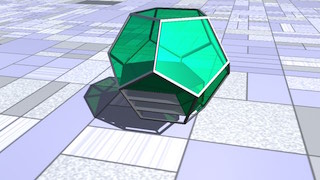• Struct Edged intersect :
Similar to Edged intersect but combines the struct of A clipped to the total volume of A B intersection
• Struct Edged subtract :
Similar to Struct subtract but the struct of A are removed when inside B, and edges are added at the intersect of Aw and Bw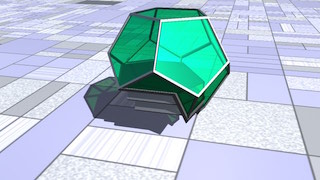##42 Min <-> Max

parameters :
A : Amplitude (0.50 : 1.50)
B : Min to Max (0. : 1.)

discussion :
Returns the minimum of the two spaces' coordinates when parameter B is 0, the maximum when B is 1 and blends the values otherwise. Result is scaled by Amplitude. The function can also be used as two
21 min max done in parallel.

Formula A* (mix( (MIN(x,z), MIN(y,w)) , (MAX(x,z), MAX(y,w)) ,B)

##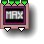42 Blended Max

parameters :
A : Balance (0. : 1.)

discussion :
This component generates a weighted maximum. The actual math is a bit complicated as the implementation guarantees continuous (smooth) output. The resulting space will have some aspects of both input spaces. The balance parameter controls whether the function gives the precise maximum or a weighted value that favors either the left or the right input pair.

Essentially, the component acts so that the left output=Max(x,z) and the right output=Max(y,w) though not strictly. With the balance set to 1, the input always wins. The effect of changes to the balance parameter can be quite surprising.

This component creates interesting juxtapositions of space.

##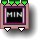42 Abs & minimum

parameters :
A : Amplitude (0.50 : 2.)
B : Rotate B (-3.14 : 3.14)

discussion :
This component provides an interesting way to combine spaces. The output is the absolute value of the minimum of x and z and y and w. Amplitude is a multiplier applied to the output. Rotate B rotates the second space before calculating the minimum.

##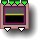42 Y compare

parameters :
A : Offset (-3.14 : 3.14)

discussion :
This is a procedural component that chooses the input pair Z or C whichever's y component has the highest value. This creates a composite space that is an interesting mix of the two inputs. The offset parameter is added to the two output values. Y Compare can also be used as a logical compare function for advanced users.

##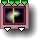42 Split space

parameters :
A : Offset (-8. : 8.)
B : Split angle (-3.14 : 3.14)

discussion :
Create a composite space whose left half comes from the left inputs and whose right half comes from the right inputs. The split is made using Space A's coordinates. So, the split might not be a straight line. The offset moves the split point left or right. Advanced users will find this useful when building fractals in iterated systems. All the split space transforms creates discontinuous spaces and are not advised for use with ArtMatic Voyager for terrains.Example file: Libraries/Components demo/Double Rotation fractal beast makes use of this function within the compiled tree.

##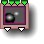42 Distance compare

parameters :
A : Offset (-8. : 8.)

discussion :
Create a composite space by picking the point from Space A or Space B that is closest to its own origin. This is another primitive that can create fascinating composite spatial systems.

##42 Split Disk

parameters :
A : Radius (0.25 : 6.28)
B : Offset x (-8. : 8.)
C : Offset y (-8. : 8.)

discussion :
This component creates a new space where space transform B (the right two inputs) is applied everywhere except the disk-shaped region in the center where space transform A is applied. Radius determines the radius of the disk in which transform A (the right two inputs) is applied.

##42 Alternate Split

parameters :
No parameters.

discussion :
Used in tandem with iterations this returns (z,w) when current iteration number is odd and (x,y) if even. So it 'alternates' space xy and zw when iterating.

##42 Split 4

parameters :
A : Offset x (0. : 16.)
B : Offset y (0. : 16.)

discussion :
Combine four circular areas drawn from space B (the two rightmost inputs) with space A (the left two inputs). This component is mostly intended for recursive systems where it can create complex fractal patterns. Parameters A & B affect the relative weight of the spaces.

##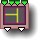42 x from Z, y from C

parameters :
No parameters.

discussion :
Creates a new space by taking the x co-ordinate from the Z input and the y coordinate from the C input. This is one of those components where the results are a bit unpredictable but often rewarding.

## 42 Compiled Tree :

parameters :
A : Amplitude
B : Recursions

discussion :
Compiled trees are groups of tiles that can be used in place of single tiles as a kind of macro or subroutine. 42 compiled tree can hold any 4 inputs / 2 outputs tree structures but is usually used for parametric or dual 2D space transform. Operations on two 2D vectors that are not built in will typically use a 4->2 CT.

usage :
Select a 42 tile and use "New compiled tree" to create a new CT from the selection (Tree Edit menu or type 'n' key). To save a CT on disk to use the function elsewhere use "Save compiled tree" from the Tree Edit menu. You may also copy and paste the entire CT by using Copy Tile and Paste Tile from the Edit menu.
42 CT can be used recursively if the option "Allow feedback" is set. In that case output is fed to the input 1 & 2 at second iteration and the transform will be applied "N" times, N being set by the "Recursions" parameter. Recursions over space transforms provide a simple an efficient way to generate 2D fractals.